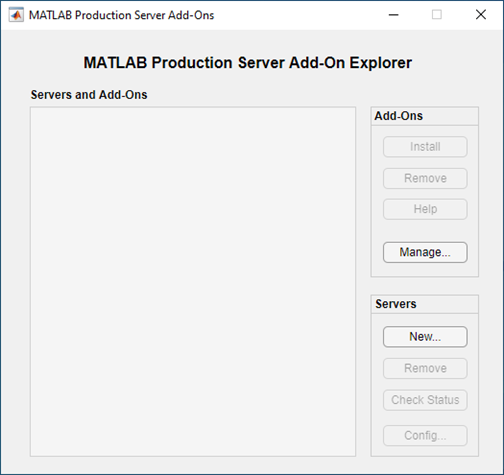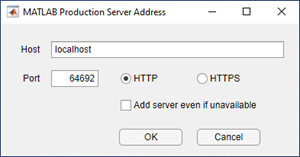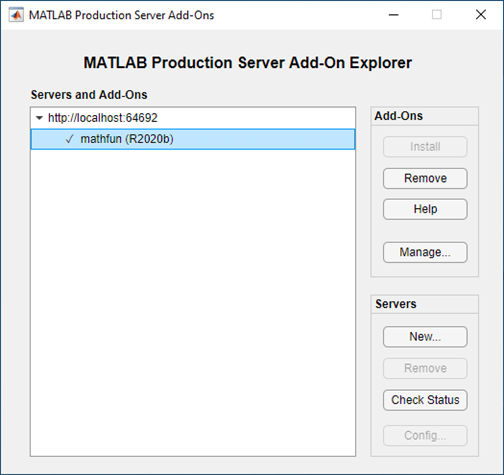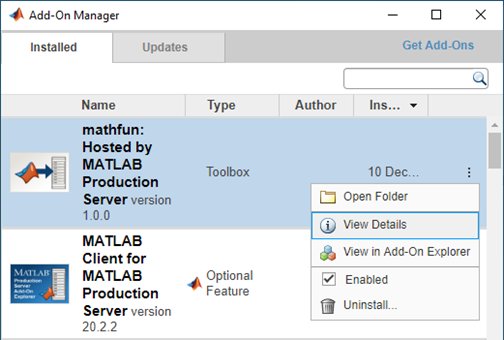Execute Deployed MATLAB Functions

This example shows how to use MATLAB® Client for MATLAB Production Server™ to invoke a MATLAB function deployed on an on-premises MATLAB Production Server instance.

MATLAB Client for MATLAB Production Server uses MATLAB Production Server add-ons to communicate between a MATLAB client and a server instance. A MATLAB Production Server add-on makes the functions in an archive deployed on MATLAB Production Server available in MATLAB. A deployed archive and its corresponding MATLAB Production Server add-on have the same name.

Installing the MATLAB Production Server add-on in your MATLAB desktop environment allows you to use the functions from a deployed archive in MATLAB. Installing a MATLAB Production Server add-on creates proxy functions of the deployed functions locally. The proxy functions manage communication between the deployed MATLAB functions and the clients that invoke the deployed functions. A proxy function and its corresponding deployed function have the same name. Since the proxy functions are MATLAB functions, you can call them from the MATLAB command prompt, other functions, or scripts. You can also compile the functions and scripts that contain the proxy functions. You can install MATLAB Production Server add-ons using the prodserver.addon.install function at the MATLAB command prompt or using the MATLAB Production Server Add-On Explorer.

Calling the proxy MATLAB function sends an HTTP request across the network to an active MATLAB Production Server instance. The server instance calls the MATLAB function in the deployable archive and passes to it the inputs from the HTTP request. The return value of the deployed MATLAB function follows the same path over the network in reverse.

The following example describes how to install MATLAB Production Server add-ons and execute a deployed MATLAB function.

Install MATLAB Client for MATLAB Production Server

Install the MATLAB Client for MATLAB Production Server support package to your MATLAB desktop environment using the MATLAB Add-On Explorer. For information about installing add-ons, see Get and Manage Add-Ons (MATLAB).

Deploy MATLAB Function on Server

1. Write a MATLAB function mymagic that uses the magic (MATLAB) function to create a magic square.

function m = mymagic(in)
m = magic(in);
end

2. Package the function mymagic in an archive named mathfun. You must include a MATLAB function signature file when you create the archive. For information about creating the function signature file, see MATLAB Function Signatures in JSON.

3. Deploy the archive mathfun on a running MATLAB Production Server instance. The server instance must have the discovery service enabled. For information about enabling the discovery service, see Discovery Service. The server administrator typically deploys the archive and configures the server.

For information on how to create and deploy the archive, see Create Deployable Archive for MATLAB Production Server and Share Deployable Archive.

Install MATLAB Production Server Add-On for the Deployable Archive

From your MATLAB desktop environment, install the MATLAB Production Server add-on for the deployed archive using the MATLAB Production Server Add-On Explorer. Installing the add-on makes the MATLAB functions deployed on the server available to your MATLAB client programs. The MATLAB Production Server Add-On Explorer is different from MATLAB Add-On Explorer.

Launch MATLAB Production Server Add-On Explorer

From a MATLAB command prompt, launch the MATLAB Production Server Add-On Explorer using the command prodserver.addon.Explorer.In the MATLAB Production Server Add-On Explorer, add information about the server that hosts the deployable archive mathfun.

1. In the Servers section, click New.

2. Enter the host name of the server in the Host box and the port number in the Port box. For example, for a server running on your local machine on port 64692, enter localhost for Host and 64692 for Port.

3. Click OK to add the server.

4. After you add the server, you can click Check Status to check the server status.After you add a server, the Servers and Add-Ons section lists the server and the MATLAB Production Server add-ons that can communicate with the server. If you add multiple servers, this sections lists all the servers and the add-ons that can communicate with each server grouped under the server that hosts them.

Install the mathfun add-on to make the MATLAB function mymagic from the deployable archive mathfun available in your MATLAB client programs.Invoke Deployed MATLAB Function

Installing an add-on creates proxy MATLAB functions locally that let you invoke MATLAB functions deployed on the server. You can call the proxy functions interactively from the MATLAB command prompt, other MATLAB functions, scripts, or standalone applications that in turn invoke the deployed MATLAB functions.

Invoke Deployed MATLAB Function from Command Line

For example, to invoke the mymagic function hosted on the server, you can call the proxy mymagic function from the matfun add-on at the MATLAB command prompt.

>> mymagic(3)

This prints a 3 by 3 magic square.

Invoke Deployed MATLAB Function from MATLAB Function

You can call the installed add-on proxy function in your MATLAB function and script. For example, write a simple MATLAB program mytranspose.m that creates a transpose of the magic square that you created using the proxy function mymagic.

function mytranspose
A = mymagic(5);
A.'
end
Running mytranspose prints the transpose of a 5 by 5 magic square.
>> mytranspose

Invoke Deployed MATLAB Function from Standalone Executable

You can call the installed add-on proxy function in your MATLAB function and then create a standalone executable from the MATLAB function. For example, you can create a standalone executable from the mytranspose MATLAB client function using MATLAB Compiler™.

>> mcc -m mytranspose

Run the standalone executable mytranspose at the system command prompt. You might need to install MATLAB Runtime if it is not installed on your machine. For more information, see MATLAB Runtime.

C:\mytranspose> mytranspose
This prints a transpose of a 5 by 5 magic square.

You can configure the standalone executable to use time out values other than the default or use a different address for the server. For more information, see Configure Client-Server Communication.

You can find more examples in the support_package_root\toolbox\mps\matlabclient\demo folder, where support_package_root is the root folder of support packages on your system. You can access the documentation by entering the doc command at the MATLAB command prompt or clicking the Help button in MATLAB desktop. In the Help browser that opens, navigate to MATLAB Client for MATLAB Production Server under Supplemental Software.